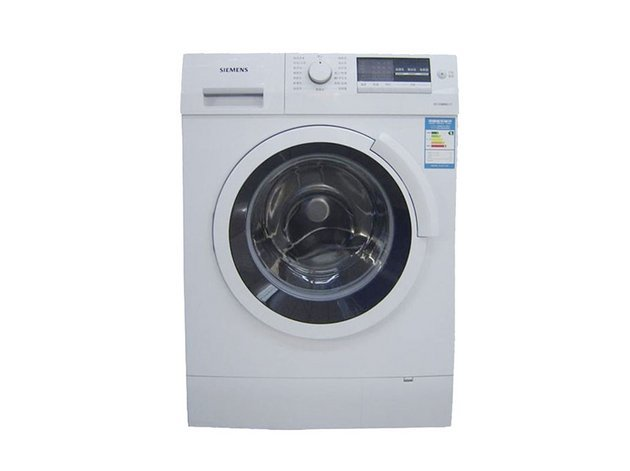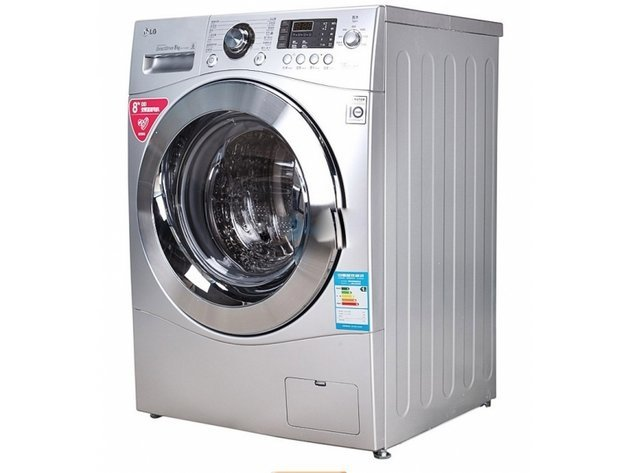|

# 滚筒洗衣机品牌 洗衣机地漏反水原因

每个人在家庭装修的时候，都希望自己的装修是最好的，每个地方都恨不得亲力亲为，让自己家的装修更加完美无缺，而洗衣机使用知识是家庭装修比较重要的一个部分，这个部分的好坏，不仅影响到整个装修的进程，还影响到以后的装修以及装修完成后的整体效果，今天，我们给大家介绍一下滚筒洗衣机品牌和洗衣机地漏反水原因，希望能给大家一些帮助。我们在使用洗衣机的同时，是要将污水排出屋外的，这时候洗衣机地漏的选择就是至关重要的了，如果洗衣机地漏没有选择好，就可能出现洗衣机地漏反水的情况，这时候洗衣机地漏反水怎么办呢？洗衣机地漏反水原因又有哪些？下面随小编看看吧。

洗衣机地漏反水怎么办及原因

第一种情况：

洗衣机地漏出现反水的情况可能是因为地漏被堵塞了，我们在使用洗衣机地漏排水时要注意避免浴室因洗头引起的头发等异物堵塞地漏的，导致水流不畅，严重的造成不排水、漫水现象。

如果是这种现象，就是要把堵塞的异物清理干净，然后把地漏装回去就可以了。在平时的时候，注意定时清理地漏里的杂物，不然很快就又会堵塞了。

第二种情况：

洗衣机要注意的是，出水管离地面过高的话，管子里面的水排不干净，长此以往集聚的水会变质产生异味，如果排水口高于洗衣机的排水电磁阀的排水口，还会影响洗衣机的正常功能。你目前出现种种问题，可以尝试把洗衣机垫高来，或是检查下水道是否堵塞。

如果是下水道堵塞，可以参考第一种方法处理。自己处理不了，可以求助于相关专业人士。

第三钟情况：

非上两种情况的地漏反水，可以试试以下的几个步骤。

第一步，我们把排水管从地漏的挂钩上取下来。

第二步，我们找个小饮料瓶，用剪刀把饮料瓶的瓶嘴剪下。

第三步，瓶嘴和排水管完美结合到一起了，严丝合缝。选择和排水管差不多大的瓶嘴是成功的关键所在。

第四步，试试排水。大多数问题都可以解决。

这里原理就是缩小排水管的内径，把它的排水量控制在地漏通过的最大流量内。

惯性思维是改进地漏，增加流量，但起码对于我们是不现实的，所以这是采取逆向思维，把重点放在排水管子上

就这样，洗衣机地漏反水完美的、简单的解决了，成本只需1毛钱。

如果您有洗衣机地漏反水的烦恼，可以按照洗衣机地漏反水怎么办的方法排查和解决。成本低，方法简单便于操作，一般的地漏反水问题大多数都可以解决。除此之外，还有另外一个方法，求助于专业人士，也很方便快捷。随着社会的发展，现在的人们大都很少自己亲手洗衣服，脏衣服一换下来，马上就扔到洗衣机里，不用多长时间就能够洗得干干净净。洗衣机的种类比较多，今天我们要讲的是其中的一种——滚筒洗衣机。那么，滚筒洗衣机哪个牌子好？滚筒洗衣机什么牌子好呢？下面我们就一起来看看2015滚筒洗衣机十大品牌排行榜吧。

滚筒洗衣机什么牌子好

1、海尔Haier ( 始于1984年,世界级家电品牌,中国企业500强,世界白色家电第一品牌,中国最具价值品牌,海尔集团公司 )

2、SIEMENS西门子 ( 始创1847年德国,世界知名家电生产商,全球极具影响力的洗衣机生产企业之一,博西家用电器(中国)有限公司 )

3、小天鹅LittleSwan ( 始于1958年,曾获中国名牌,美的集团旗下,上市公司,最具市场竞争力品牌之一,无锡小天鹅股份有限公司 )

4、松下洗衣机 ( 于1918年日本,世界500强企业,世界品牌,大型跨国公司,全球最大的电子厂商之一,杭州松下家用电器有限公司 )

5、SANYO三洋 ( 于1947年日本,惠而浦中国控股合肥三洋变更而来,上市公司,惠而浦(中国)股份有限公司 )

滚筒洗衣机什么牌子好

6、LG洗衣机 ( 于1947年韩国,世界著名洗衣机品牌,世界500强韩国LG与熊猫电子集团共同组建,南京乐金熊猫电器有限公司 )

7、SAMSUNG三星 ( 始于1938年韩国,世界500强企业,全球消费电子领域龙头企业,世界著名跨国公司,三星集团 )

8、Whirlpool惠而浦 ( 创立于1911年,全球最具规模的大型白色家电制造商之一,世界顶级家电生产商和零售商,惠而浦(中国)股份有限公司 )

9、美的Midea ( 始于1981年,世界级的白色家电制造商和品牌商,上市公司,中国企业500强,美的集团股份有限公司 )

10、BOSCH博世 ( 世界知名品牌,欧洲家电市场的领先品牌,中国知名品牌,行业影响力品牌,博西家用电器(中国)有限公司 )

以上是小编给大家介绍的关于洗衣机使用知识的信息，如果大家还想要了解关于冰箱使用知识的信息，就请多多关注我们，将给大家提供更全面、更详细、更新的资讯信息。想了解更多关于洗衣机的特价信息，请关注每周末的建材家具团购会，价格将低于市场30%-50%，了解更多洗衣机特价请搜索进入。

`声明：本文由入驻焦点开放平台的作者撰写，除焦点官方账号外，观点仅代表作者本人，不代表焦点立场错误信息举报电话： 400-099-0099，邮箱：jubao@vip.sohu.com，或点此进行意见反馈，或点此进行举报投诉。`A B C D E F G H J K L M N P Q R S T W X Y Z
A - B - C - D - E
• A
• 鞍山
• 安庆
• 安阳
• 安顺
• 安康
• 澳门
• B
• 北京
• 保定
• 包头
• 巴彦淖尔
• 本溪
• 蚌埠
• 亳州
• 滨州
• 北海
• 百色
• 巴中
• 毕节
• 保山
• 宝鸡
• 白银
• 巴州
• C
• 承德
• 沧州
• 长治
• 赤峰
• 朝阳
• 长春
• 常州
• 滁州
• 池州
• 长沙
• 常德
• 郴州
• 潮州
• 崇左
• 重庆
• 成都
• 楚雄
• 昌都
• 慈溪
• 常熟
• D
• 大同
• 大连
• 丹东
• 大庆
• 东营
• 德州
• 东莞
• 德阳
• 达州
• 大理
• 德宏
• 定西
• 儋州
• 东平
• E
• 鄂尔多斯
• 鄂州
• 恩施
F - G - H - I - J
• F
• 抚顺
• 阜新
• 阜阳
• 福州
• 抚州
• 佛山
• 防城港
• G
• 赣州
• 广州
• 桂林
• 贵港
• 广元
• 广安
• 贵阳
• 固原
• H
• 邯郸
• 衡水
• 呼和浩特
• 呼伦贝尔
• 葫芦岛
• 哈尔滨
• 黑河
• 淮安
• 杭州
• 湖州
• 合肥
• 淮南
• 淮北
• 黄山
• 菏泽
• 鹤壁
• 黄石
• 黄冈
• 衡阳
• 怀化
• 惠州
• 河源
• 贺州
• 河池
• 海口
• 红河
• 汉中
• 海东
• 怀来
• I
• J
• 晋中
• 锦州
• 吉林
• 鸡西
• 佳木斯
• 嘉兴
• 金华
• 景德镇
• 九江
• 吉安
• 济南
• 济宁
• 焦作
• 荆门
• 荆州
• 江门
• 揭阳
• 金昌
• 酒泉
• 嘉峪关
K - L - M - N - P
• K
• 开封
• 昆明
• 昆山
• L
• 廊坊
• 临汾
• 辽阳
• 连云港
• 丽水
• 六安
• 龙岩
• 莱芜
• 临沂
• 聊城
• 洛阳
• 漯河
• 娄底
• 柳州
• 来宾
• 泸州
• 乐山
• 六盘水
• 丽江
• 临沧
• 拉萨
• 林芝
• 兰州
• 陇南
• M
• 牡丹江
• 马鞍山
• 茂名
• 梅州
• 绵阳
• 眉山
• N
• 南京
• 南通
• 宁波
• 南平
• 宁德
• 南昌
• 南阳
• 南宁
• 内江
• 南充
• P
• 盘锦
• 莆田
• 平顶山
• 濮阳
• 攀枝花
• 普洱
• 平凉
Q - R - S - T - W
• Q
• 秦皇岛
• 齐齐哈尔
• 衢州
• 泉州
• 青岛
• 清远
• 钦州
• 黔南
• 曲靖
• 庆阳
• R
• 日照
• 日喀则
• S
• 石家庄
• 沈阳
• 双鸭山
• 绥化
• 上海
• 苏州
• 宿迁
• 绍兴
• 宿州
• 三明
• 上饶
• 三门峡
• 商丘
• 十堰
• 随州
• 邵阳
• 韶关
• 深圳
• 汕头
• 汕尾
• 三亚
• 三沙
• 遂宁
• 山南
• 商洛
• 石嘴山
• T
• 天津
• 唐山
• 太原
• 通辽
• 铁岭
• 泰州
• 台州
• 铜陵
• 泰安
• 铜仁
• 铜川
• 天水
• 天门
• W
• 乌海
• 乌兰察布
• 无锡
• 温州
• 芜湖
• 潍坊
• 威海
• 武汉
• 梧州
• 渭南
• 武威
• 吴忠
• 乌鲁木齐
X - Y - Z
• X
• 邢台
• 徐州
• 宣城
• 厦门
• 新乡
• 许昌
• 信阳
• 襄阳
• 孝感
• 咸宁
• 湘潭
• 湘西
• 西双版纳
• 西安
• 咸阳
• 西宁
• 仙桃
• 西昌
• Y
• 运城
• 营口
• 盐城
• 扬州
• 鹰潭
• 宜春
• 烟台
• 宜昌
• 岳阳
• 益阳
• 永州
• 阳江
• 云浮
• 玉林
• 宜宾
• 雅安
• 玉溪
• 延安
• 榆林
• 银川
• Z
• 张家口
• 镇江
• 舟山
• 漳州
• 淄博
• 枣庄
• 郑州
• 周口
• 驻马店
• 株洲
• 张家界
• 珠海
• 湛江
• 肇庆
• 中山
• 自贡
• 资阳
• 遵义
• 昭通
• 张掖
• 中卫

1室1厅1厨1卫1阳台

1
2
3
4
5

0
1
2

1

1

0
1
2
3报名成功，资料已提交审核A B C D E F G H J K L M N P Q R S T W X Y Z
A - B - C - D - E
• A
• 鞍山
• 安庆
• 安阳
• 安顺
• 安康
• 澳门
• B
• 北京
• 保定
• 包头
• 巴彦淖尔
• 本溪
• 蚌埠
• 亳州
• 滨州
• 北海
• 百色
• 巴中
• 毕节
• 保山
• 宝鸡
• 白银
• 巴州
• C
• 承德
• 沧州
• 长治
• 赤峰
• 朝阳
• 长春
• 常州
• 滁州
• 池州
• 长沙
• 常德
• 郴州
• 潮州
• 崇左
• 重庆
• 成都
• 楚雄
• 昌都
• 慈溪
• 常熟
• D
• 大同
• 大连
• 丹东
• 大庆
• 东营
• 德州
• 东莞
• 德阳
• 达州
• 大理
• 德宏
• 定西
• 儋州
• 东平
• E
• 鄂尔多斯
• 鄂州
• 恩施
F - G - H - I - J
• F
• 抚顺
• 阜新
• 阜阳
• 福州
• 抚州
• 佛山
• 防城港
• G
• 赣州
• 广州
• 桂林
• 贵港
• 广元
• 广安
• 贵阳
• 固原
• H
• 邯郸
• 衡水
• 呼和浩特
• 呼伦贝尔
• 葫芦岛
• 哈尔滨
• 黑河
• 淮安
• 杭州
• 湖州
• 合肥
• 淮南
• 淮北
• 黄山
• 菏泽
• 鹤壁
• 黄石
• 黄冈
• 衡阳
• 怀化
• 惠州
• 河源
• 贺州
• 河池
• 海口
• 红河
• 汉中
• 海东
• 怀来
• I
• J
• 晋中
• 锦州
• 吉林
• 鸡西
• 佳木斯
• 嘉兴
• 金华
• 景德镇
• 九江
• 吉安
• 济南
• 济宁
• 焦作
• 荆门
• 荆州
• 江门
• 揭阳
• 金昌
• 酒泉
• 嘉峪关
K - L - M - N - P
• K
• 开封
• 昆明
• 昆山
• L
• 廊坊
• 临汾
• 辽阳
• 连云港
• 丽水
• 六安
• 龙岩
• 莱芜
• 临沂
• 聊城
• 洛阳
• 漯河
• 娄底
• 柳州
• 来宾
• 泸州
• 乐山
• 六盘水
• 丽江
• 临沧
• 拉萨
• 林芝
• 兰州
• 陇南
• M
• 牡丹江
• 马鞍山
• 茂名
• 梅州
• 绵阳
• 眉山
• N
• 南京
• 南通
• 宁波
• 南平
• 宁德
• 南昌
• 南阳
• 南宁
• 内江
• 南充
• P
• 盘锦
• 莆田
• 平顶山
• 濮阳
• 攀枝花
• 普洱
• 平凉
Q - R - S - T - W
• Q
• 秦皇岛
• 齐齐哈尔
• 衢州
• 泉州
• 青岛
• 清远
• 钦州
• 黔南
• 曲靖
• 庆阳
• R
• 日照
• 日喀则
• S
• 石家庄
• 沈阳
• 双鸭山
• 绥化
• 上海
• 苏州
• 宿迁
• 绍兴
• 宿州
• 三明
• 上饶
• 三门峡
• 商丘
• 十堰
• 随州
• 邵阳
• 韶关
• 深圳
• 汕头
• 汕尾
• 三亚
• 三沙
• 遂宁
• 山南
• 商洛
• 石嘴山
• T
• 天津
• 唐山
• 太原
• 通辽
• 铁岭
• 泰州
• 台州
• 铜陵
• 泰安
• 铜仁
• 铜川
• 天水
• 天门
• W
• 乌海
• 乌兰察布
• 无锡
• 温州
• 芜湖
• 潍坊
• 威海
• 武汉
• 梧州
• 渭南
• 武威
• 吴忠
• 乌鲁木齐
X - Y - Z
• X
• 邢台
• 徐州
• 宣城
• 厦门
• 新乡
• 许昌
• 信阳
• 襄阳
• 孝感
• 咸宁
• 湘潭
• 湘西
• 西双版纳
• 西安
• 咸阳
• 西宁
• 仙桃
• 西昌
• Y
• 运城
• 营口
• 盐城
• 扬州
• 鹰潭
• 宜春
• 烟台
• 宜昌
• 岳阳
• 益阳
• 永州
• 阳江
• 云浮
• 玉林
• 宜宾
• 雅安
• 玉溪
• 延安
• 榆林
• 银川
• Z
• 张家口
• 镇江
• 舟山
• 漳州
• 淄博
• 枣庄
• 郑州
• 周口
• 驻马店
• 株洲
• 张家界
• 珠海
• 湛江
• 肇庆
• 中山
• 自贡
• 资阳
• 遵义
• 昭通
• 张掖
• 中卫• 手机• 分享
• 设计
免费设计
• 计算器
装修计算器
• 入驻
合作入驻
• 联系
联系我们
• 置顶
返回顶部